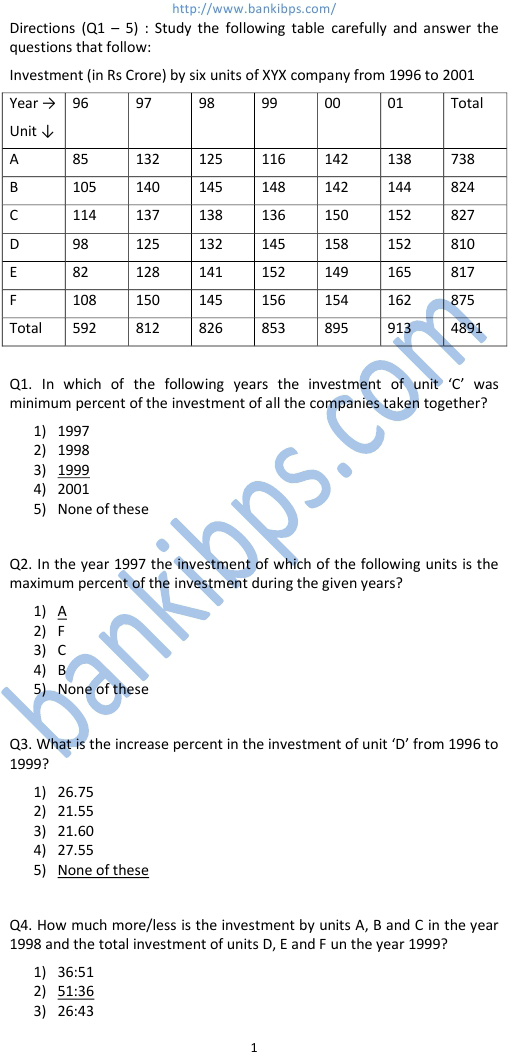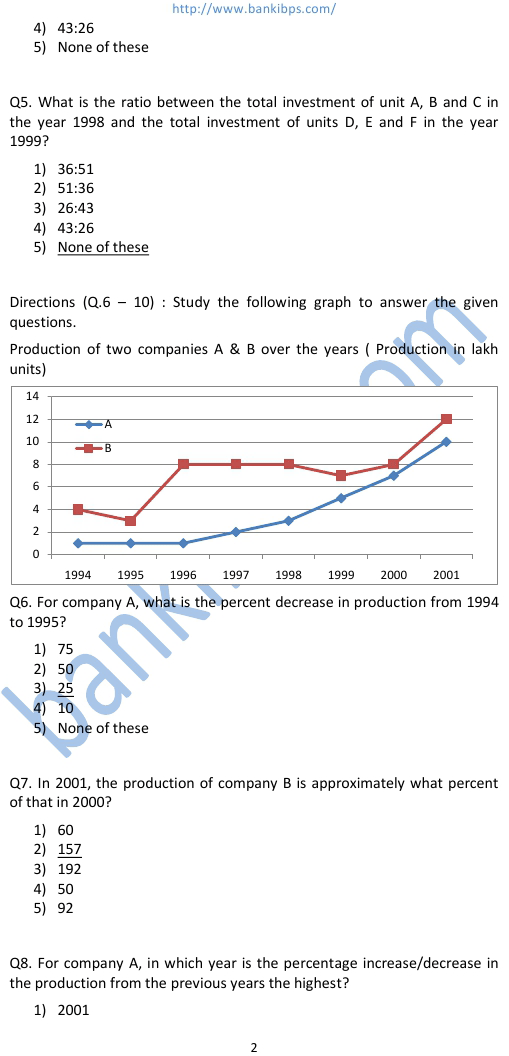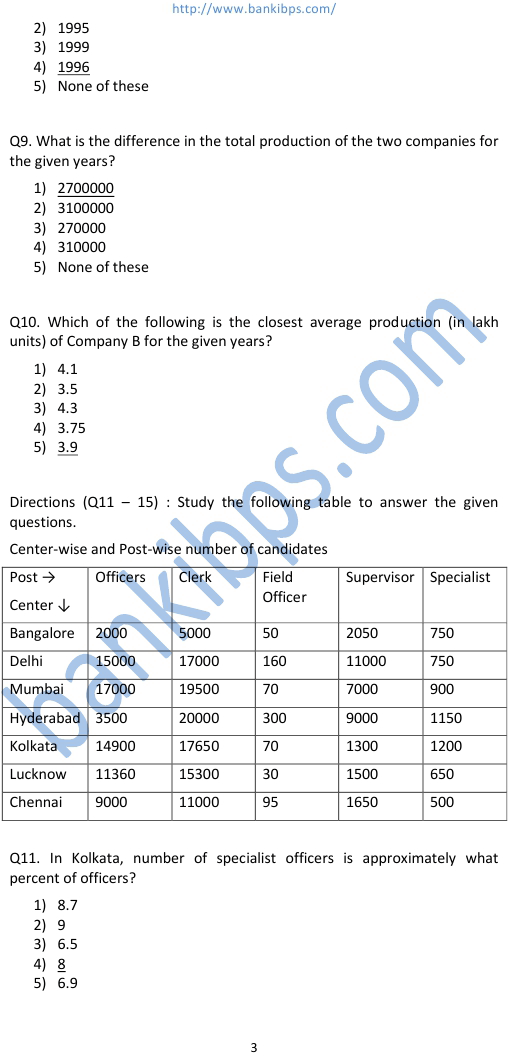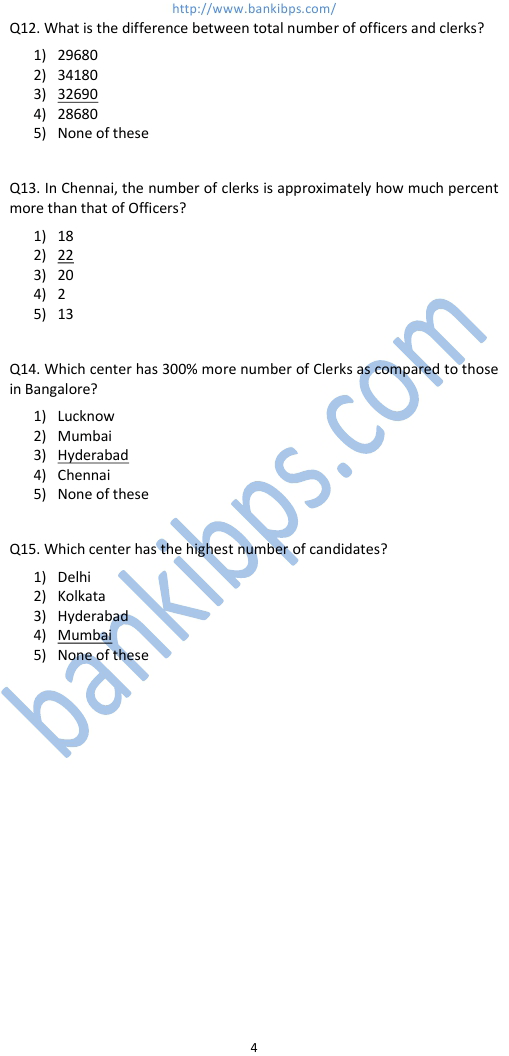# Data Interpretation Practice

Q1. In which of the following years the investment of unit ‘C’ was minimum percent of the investment of all the data interpretation practice companies taken together? 1) 1997 2) 1998 3) 1999 4) 2001 5) None of these Q2. In the year 1997 the investment of which of the following units is the maximum percent of the investment during the given years? 1) A 2) F 3) C 4) B 5) None of these Q3. What is the increase percent in the investment of unit ‘D’ from 1996 to 1999? 1) 26.75 2) 21.55 3) 21.60 4) 27.55 5) None of these Q4. How much more/less is the investment by units A, B and C in the year 1998 and the total investment of units D, E and F un the year 1999? 1) 36:51 2) 51:36 3) 26:43
Practice Exercise - 203 [ Data Interpretation ]## data interpretation practice

### data interpretation practice test

#### data interpretation practice questions

##### data interpretation practice test pdf
###### write data interpretation
gre data interpretation practice. data interpretation practice test. data interpretation practice questions. data interpretation gre practice questions. data interpretation practice cat. data interpretation practice gre. data interpretation practice test pdf. data interpretation practice questions cat. data interpretation problems. write data interpretation. format for data interpretation.